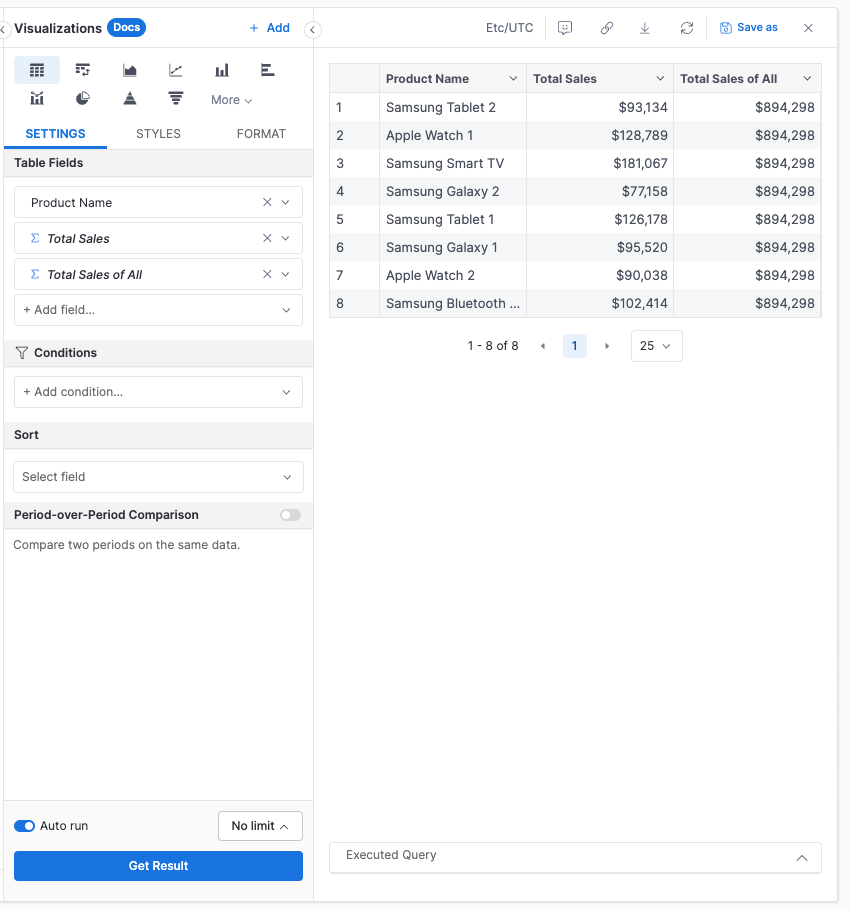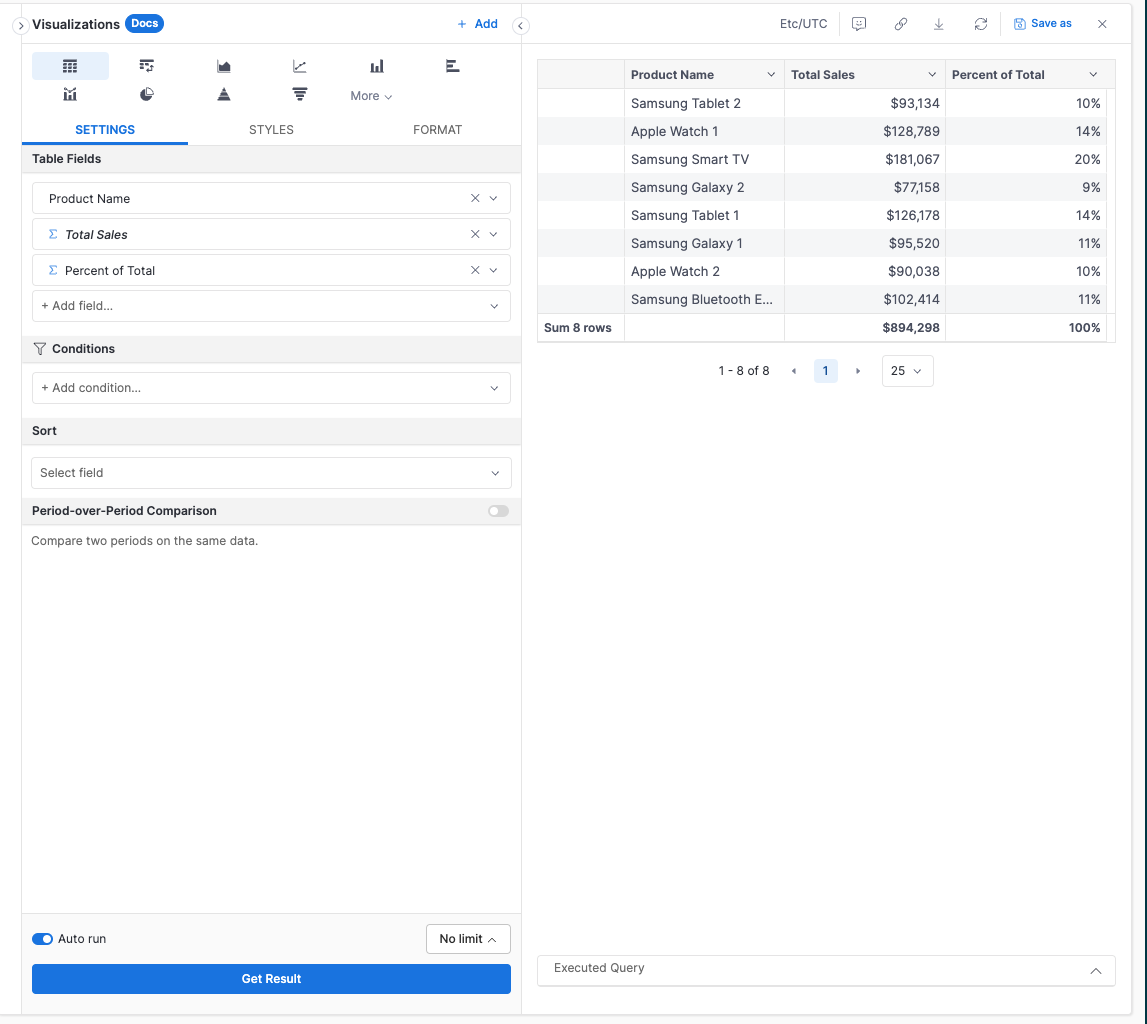# Percent of Total

info

This is part of our beta expression language AQL. Learn more. Request beta.

## Introduction​

The Percent of Total analysis is a commonly used reporting technique that helps understand the contribution of each row value to the overall populatiion.

In SQL, you have to divide your calculation into multiple CTEs (Common Table Expressions) and then cross-join those together to perform Percent of Total. Whereas with AQL, you can achieve this calculation in a more concise and reusable way by focusing on the business logic rather than the technical semantics.

## High-level Flow​

Suppose we want to see how each product’s sales contribute to global sales.To illustrate the calculation using AQL, we’ll explore two different ways of performing it. First, we will break down the calculation into manageable steps or components. Then, we'll demonstrate how to write it as a single-line query.

## Setup​

To make it simple, we'll be working on the order_items modeling definition only with the following fields.

Model order_items {  ...    dimension id {...}  dimension product {...}  dimension amount {...}}

## Implementation​

1. Calculate the total sales value for each product by defining the measure total_sales

Model order_items {  ...    measure total_sales {    label: 'Total Sales'    type: 'number'    definition: @aql sum(order_items.amount) ;;  }}1. Total sales of all order items is also needed to be used as denominator for the percent of total. This measure can be definied by using of_all() macro.

This macro calculates sum(amount) on all order items while remainaining constant when this measure is reused for further calculations. We'll get into how "remain constant" looks like at the very next step

Model order_items {  ...  measure total_sales {...}  measure _total_sales_of_all {    label: 'Total Sales of All'    type: 'number'    definition: @aql sum(order_items.amount) | of_all(order_items.id) ;;  }}1. Finally, the Percent of Total is determined by dividing the total_sales by _total_sales_of_all. Since _total_sales_of_all remains constant, the percent of total will adjust according to total_sales measure and its corresponding dimension

Model order_items {  measure total_sales {...}  measure _total_sales_of_all {...}  measure percent_of_total {    label: "Percent of Total"    type: 'number'    definition: @aql (order_items.total_sales*1.0) / order_items._total_sales_of_all ;;  }}

The final result will look like belowThe entire steps described above can also be straightforwardly condensed into one single-line expression.

Model order_items {  measure percent_of_total {    label: "Percent of Total"    type: 'number'    definition: @aql (sum(order_items.amount) * 1.0) / (sum(order_items.amount) | of_all(order_items));;  }}

To maintain the decimal format for the percent of total measure, multiplying by 1.0 explicitly casts the left side to decimal.